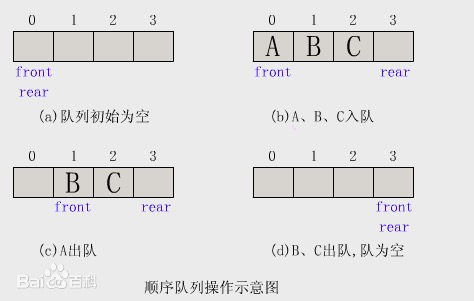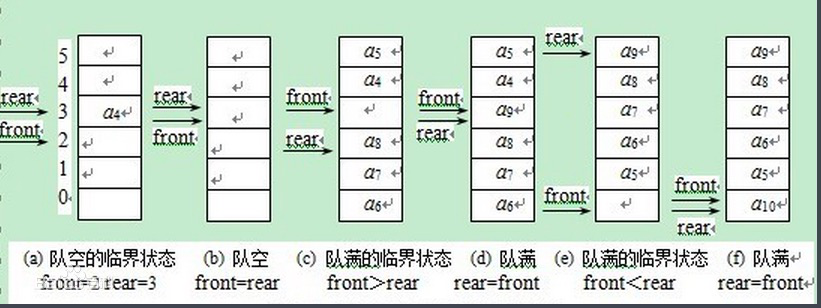# 队列的定义及模拟实现

### 顺序队列#### 实现（数组）

function ArrayQueue(){
var arr = [];
//入队操作
this.push = function(element){
arr.push(element);
return true;
}
//出队操作
this.pop = function(){
return arr.shift();
}
//获取队首
this.getFront = function(){
return arr;
}
//获取队尾
this.getRear = function(){
return arr[arr.length - 1]
}
//清空队列
this.clear = function(){
arr = [];
}
//获取队长
this.size = function(){
return length;
}
} 

### 循环队列#### 实现（ES6）

class MyCircularQueue {
constructor(size) {
this.size = size;
this.queue = [];
}
enQueue(value) {
if (this.isFull()) return false;
this.queue.push(value);
return true;
}
deQueue() {
if (this.isEmpty()) return false;
this.queue.shift();
return true;
}
Front() {
if (this.isEmpty()) return -1;
return this.queue;
}
Rear() {
if (this.isEmpty()) return -1;
return this.queue[this.queue.length - 1];
}
isEmpty() {
return this.queue.length === 0;
}
isFull() {
return this.queue.length === this.size;
}
}

posted @ 2020-09-02 19:52  一只菜鸟攻城狮啊  阅读(32)  评论(0编辑  收藏